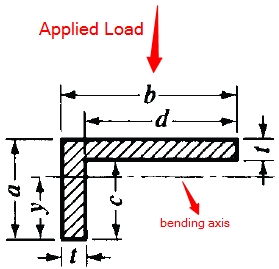This is the L Beam Moment of Inertia Calculator. Simply enter the dimensions below for L beam to find the moment of inertia.d = c = b = t = a =